#Euler-Cauchy EquationsAn Euler-Cauchy equation is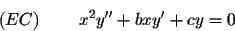where b and c are constant numbers. Let us consider the change of variable

x = et.

Then we have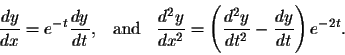The equation (EC) reduces to the new equation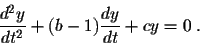We recognize a second order differential equation with constant coefficients. Therefore, we use the previous sections to solve it. We summarize below all the cases:
(1)
Write down the characteristic equation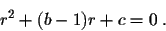(2)
If the roots r1 and r2 are distinct real numbers, then the general solution of (EC) is given by

y(x) = c1 |x|r1 + c2 |x|r2.

(2)
If the roots r1 and r2 are equal (r1 = r2), then the general solution of (EC) is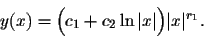(3)
If the roots r1 and r2 are complex numbers, then the general solution of (EC) is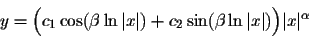where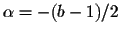and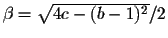.

Example: Find the general solution to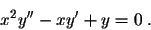Solution: First we recognize that the equation is an Euler-Cauchy equation, with b=-1 and c=1.
1
Characteristic equation is r2 -2r + 1=0.
2
Since 1 is a double root, the general solution is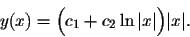[Differential Equations] [First Order D.E.] [Second Order D.E.]
[Geometry] [Algebra] [Trigonometry ]
[Calculus] [Complex Variables] [Matrix Algebra]S.O.S MATHematics home page

Do you need more help? Please post your question on our S.O.S. Mathematics CyberBoard.Author: Mohamed Amine Khamsi